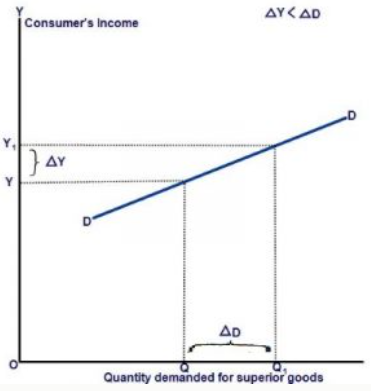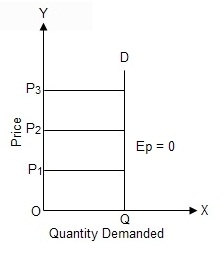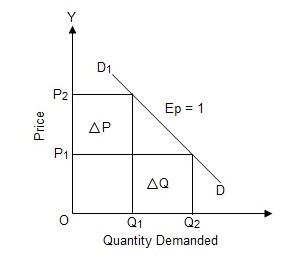1. The proportionate change in quantity demanded for a goods due to the proportionate change in consumer's income is called income elasticity of demand. It is usually written as:There are three types of income elasticity:
1. Positive Income Elasticity :
If increase in income leads to increase in demand of a commodity and decrease in income leads to decrease in demand of a commodity, it is called positive income elasticity. Positive income elasticity can be divided into  the following three types:

a. Income elasticity greater than unity:
If the percentage change in quantity demanded for a commodity is greater than percentage change in income of the consumer, it is said to be income  elasticity greater than unity.In the given figure, quantity demanded and consumer’s income is measured along X-axis and Y-axis respectively. The small rise in income from OY to OY1 has caused greater rise in the quantity demanded from OQ to OQ1 and vice versa. Thus, the demand curve DD shows income elasticity greater than unity.

b. Income elasticity less than unity:

If the percentage change in quantity demanded for a commodity is less than percentage change in income of the consumer, it is said to be income elasticity less than unity.In the given figure, quantity demanded and consumer’s income is measured along X-axis and Y-axis respectively. The greater rise in income from OY to OY1 has caused small rise in the quantity demanded from OQ to OQ1 and vice versa. Thus, the demand curve DD shows income elasticity less than unity.

c. Income elasticity equal to unity:

If the percentage change in quantity demanded for a commodity is equal to percentage change in income of the consumer, it is said to be income elasticity equal to unity.In the given figure, quantity demanded and consumer’s income is measured along X-axis and Y-axis respectively. The small rise in income from OY to OY1 has caused equal rise in the quantity demanded from OQ to OQ1 and vice versa. Thus, the demand curve DD shows income elasticity equal to unity.

2. Zero Income Elasticity:

If the quantity demanded for a commodity remains constant with any rise or fall in income of the consumer and, it is said to be zero income elasticity of demand.In the given figure, quantity demanded and consumer’s income is measured along X-axis and Y-axis respectively. The consumer’s income may fall to OY1 or rise to OY2 from OY, the quantity demanded remains the same at OQ. Thus, the demand curve DD, which is vertical straight line parallel to Y-axis shows zero income elasticity of demand.

3. Negative Income Elasticity:

If there is inverse relationship between income of the consumer and demand for the commodity, then income elasticity will be negative. That is, if the quantity demanded for a commodity decreases with the rise in income of the consumer and vice versa, it is said to be negative income elasticity of demand.In the given figure, quantity demanded and consumer’s income is measured along X-axis and Y-axis respectively. When the consumer’s income rises from OY to OY1 the quantity demanded of inferior goods falls from OQ to OQ1  and vice versa. Thus, the demand curve DD shows negative income elasticity of demand.

2. The determinants of price elasticity of demand are as follow:
1.Substitute goods :
If a good has many substitutes, a change in its price will have a major impact on its demand. Consumers will  turn to the substitute goods instead of buying a good that suddenly has become more expensive. A good that has many substitutes thus also has a high price elasticity of demand.

2. Proportion of income:
The larger proportion of income a good constitutes, the more responsive its consumers will be to changes in price. If a good only constitutes a small proportion of a consumer's income, the demand for the good is likely to be price inelastic.

3.Luxury or necessity:
I
f a good is a necessity (eg. food), consumers will continue to buy it regardless of changes in price. If a good is a luxury good, an increase in price might cause consumers to stop buying the good. Thus, the demand for luxury goods tends to be price elastic, while the demand for necessity goods is price inelastic.

M
uch like necessity goods, if a good is addictive (eg. cigarettes), consumers will continue to buy it despite changes in price - its demand is price inelastic. If a good is not addictive, consumers will be more responsible to changes in its price.

5.Time to respond :
In the long term, the demand for most goods is price elastic. This is because over time, consumers can find (cheaper) substitute goods and quit addictions - in the short time they cannot. If a change in price is only temporary, costumers are unlikely to bother doing this. Hence, in the short term, demand is rather price inelastic.

3. The percentage change in quantity demanded of a commodity due to percentage change in price of that commodity is known as price elasticity of demand.There are 5 types of price elasticity of demand.

1.  Perfectly Elastic Demand:
When negligible change in price leads to infinite change in quantity demanded ,the demand for a commodity is said to be perfectly elastic demand.In the given figure, the price is measured in OY-axis and quantity demanded is measured along the OX-axis. PD is the demand curve which is parallel to OX-axis. Perfectly elastic demand shows that quantity demanded for a goods increases infinitely with a small fall in price or becomes zero with a small rise in price.

2.       Perfectly Inelastic Demand:
When the quantity demanded does not change with the change in its price, the demand for a commodity is said to perfectly inelastic demand.In the given figure, the price is measured in OY-axis and quantity demanded is measured along the OX-axis. QD is the demand curve which is parallel to OY-axis. Here, whatever be the price either OP1 or OP2 or OP quantity demanded is same as OQ.

3.       Unitary Elastic Demand:
When the percentage change in quantity demanded is equal to the percentage change in price of  commodity it is said to be unitary elastic demand.In the given figure, the price is measured along OY-axis and quantity demanded is measured along the OX-axis. D1D is the demand curve which is unitary elastic, Here, the increase in quantity demanded for a goods from OQto OQ2 equal to the decrease in price of goods from OPto OP1. So, it indicates unitary elastic demand.

4.      Relatively Elastic Demand :
When the percentage change in the quantity  demanded for a commodity is more than percentage change in its price, it is called relatively elastic demand.In the given figure, the price is measured along OY-axis and quantity demanded is measured along OX-axis. D1D is the demand curve which is relatively elastic. Here, increase in quantity demanded from OQ1 to OQ2 is more than the decrease in price from OP2 to OP1Thus, it indicates relatively elastic demand.

5.    Relatively Inelastic Demand:
When the percentage change in the quantity demanded of a commodity is less than the percentage change in its     price, it is called relatively inelastic demand.In the given figure, the price is measured along OY-axis and quantity demanded is measured along the OX-axis. D1D is the demand curve which is relatively inelastic. Here, the increase in quantity demanded for a goods from OQto OQis less than the decreases in price of the goods from OP2 to OP1. So, it indicates relatively inelastic demand.

There are no MCQs in Mattrab Library for this chapter yet.